# Statistics review 1: Presenting and summarising data

## Abstract

The present review is the first in an ongoing guide to medical statistics, using specific examples from intensive care. The first step in any analysis is to describe and summarize the data. As well as becoming familiar with the data, this is also an opportunity to look for unusually high or low values (outliers), to check the assumptions required for statistical tests, and to decide the best way to categorize the data if this is necessary. In addition to tables and graphs, summary values are a convenient way to summarize large amounts of information. This review introduces some of these measures. It describes and gives examples of qualitative data (unordered and ordered) and quantitative data (discrete and continuous); how these types of data can be represented figuratively; the two important features of a quantitative dataset (location and variability); the measures of location (mean, median and mode); the measures of variability (range, interquartile range, standard deviation and variance); common distributions of clinical data; and simple transformations of positively skewed data.

## Introduction

Data description is a vital part of any research project and should not be ignored in the rush to start testing hypotheses. There are many reasons for this important process, such as gaining familiarity with the data, looking for unusually high or low values (outliers) and checking the assumptions required for statistical testing. The two most common types of data are qualitative and quantitative (Fig. 1). Qualitative data fall into two categories: unordered qualitative data, such as ventilatory support (none, non-invasive, intermittent positive-pressure ventilation, oscillatory); and ordered qualitative data, such as severity of disease (mild, moderate, severe). Quantitative data are numerical and fall into two categories: discrete quantitative data, such as the number of days spent in intensive care; and continuous quantitative data, such as blood pressure or haemoglobin concentrations. Tables are a useful way of describing both qualitative and grouped quantitative data and there are also many types of graph that provide a convenient summary. Qualitative data are commonly described using bar or pie charts, whereas quantitative data can be represented using histograms or box and whisker plots.

Tables and graphs provide a convenient simple picture of a set of data (dataset), but it is often necessary to further summarize quantitative data, for example for hypothesis testing. The two most important elements of a dataset are its location (where on average the data lie) and its variability (the extent to which individual data values deviate from the location). There are several different measures of location and variability that can be calculated, and the choice of which to use depends on individual circumstances.

## Measuring location

### Mean

The mean is the most well known average value. It is calculated by summing all of the values in a dataset and dividing them by the total number of values. The algebraic notation for the mean of a set of n values (X1, X2,...,Xn) is: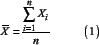where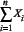is the mathematical notation for the sum of all values (X1, X2,...,Xn). In other words: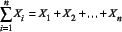Of all the measures of location, the mean is the most commonly used because it is easily understood and has useful mathematical properties that make it convenient for use in many statistical contexts. It is strongly influenced by extreme values (outliers), however, and is most representative when the data are symmetrically distributed (see below).

### Median

The median is the central value when all observations are sorted in order. If there is an odd number of observations then it is simply the middle value; if there is an even number of observations then it is the average of the middle two. The median does not have the beneficial mathematical properties of the mean. However, it is not generally influenced by extreme values (outliers), and as a result it is particularly useful in situations where there are unusually low or high values that would render the mean unrepresentative of the data.

### Mode

The mode is simply the most commonly occurring value in the data. It is not generally used because it is often not representative of the data, particularly when the dataset is small.

### Example of calculating location

To see how these quantities are calculated in practise, consider the data shown in Table 1. These are haemoglobin concentration measurements taken from 48 patients on admission to an intensive care unit, listed here in ascending order.

The first step in exploring these data is to construct a histogram to illustrate the shape of the distribution. Rather than plot the frequency of each value separately (e.g. one patient with haemoglobin 5.4 g/dl, two patients with haemoglobin 6.4 g/dl, one patient with haemoglobin 7.0 g/dl, and so on), continuous data are generally grouped or categorized before plotting (e.g. one patient with haemoglobin between 5.0 and 5.9 g/dl, two patients with haemoglobin between 6.0 and 6.9 g/dl, four patients with haemoglobin between 7.0 and 7.9 g/dl, and so on). These categories can be defined in any way and need not necessarily be of the same width, although it is generally more convenient to have equally sized groups. However, the categories must be exhaustive (the categories must cover the full range of values in the dataset) and exclusive (there should be no overlap between categories). Therefore, if one category ends with 6.9 g/dl then the next must begin with 7.0 g/dl rather than 6.9 g/dl. Fig. 2 shows the data in Table 1 grouped into 1 g/dl categories (5.0-5.9, 6.0-6.9,...,14.0-14.9 g/dl).

Fig. 2 shows that the data are roughly symmetrically distributed; more common values are clustered around a peak in the middle of the distribution, with decreasing numbers of smaller and larger values on either side. The mean, median and mode of these data are shown in Table 2.

Notice that the mean and the median are similar. This is because the data are approximately symmetrical. In general, the mean, median and mode will be similar in a dataset that has a symmetrical distribution with a single peak, such as that shown in Fig. 2. However, the dataset presented here is rather small and so the mode is not such a good measure of location.

## Measuring variability

### Range

As with location, there are a number of different measures of variability. The simplest of these is probably the range, which is the difference between the largest and smallest observation in the dataset. The disadvantage of this measure is that it is based on only two of the observations and may not be representative of the whole dataset, particularly if there are outliers. In addition, it gives no information regarding how the data are distributed between the two extremes.

### Interquartile range

An alternative to the range is the interquartile range. Quartiles are calculated in a similar way to the median; the median splits a dataset into two equally sized groups, tertiles split the data into three (approximately) equally sized groups, quartiles into four, quintiles into five, and so on. The interquartile range is the range between the bottom and top quartiles, and indicates where the middle 50% of the data lie. Like the median, the interquartile range is not influenced by unusually high or low values and may be particularly useful when data are not symmetrically distributed. Ranges based on alternative subdivisions of the data can also be calculated; for example, if the data are split into deciles, 80% of the data will lie between the bottom and top deciles and so on.

### Standard deviation

The standard deviation is a measure of the degree to which individual observations in a dataset deviate from the mean value. Broadly, it is the average deviation from the mean across all observations. It is calculated by squaring the difference of each individual observation from the mean (squared to remove any negative differences), adding them together, dividing by the total number of observations minus 1, and taking the square root of the result.

Algebraically the standard deviation for a set of n values (X1,X2,...,Xn} is written as follows: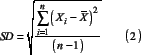where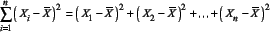and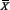is the mean described above (Eqn 1). It can be seen from this expression that if individual observations are all close to the mean then the standard deviation will be small (at the extreme, if all observations were equal to the mean then the standard deviation would be zero). Conversely, if the observations vary widely then the standard deviation will be substantially larger. The standard deviation summarizes a great deal of information in one number and, like the mean, has useful mathematical properties.

### Variance

Another measure of variability that may be encountered is the variance. This is simply the square of the standard deviation: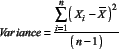The variance is not generally used in data description but is central to analysis of variance (covered in a subsequent review in this series).

### Example of calculating variability

Table 3 shows the calculation of the range, interquartile range and standard deviation of the data shown in Table 1. The range, from 5.4 to 14.1 g/dl, indicates the full extent of the data, but does not give any information regarding how the remaining observations are distributed between these extremes. For example, it may be that the lower value of 5.4 g/dl is an outlier and the remainder of the observations are all over 10.0 g/dl, or that most values lie at the lower end of the range with substantially fewer at the other extreme. It is impossible to tell this from the range alone.

The interquartile range (which contains the central 50% of the data) gives a better indication of the general shape of the distribution, and indicates that 50% of all observations fall in a rather narrower range (from 8.7 to 10.8 g/dl). In addition, the median and mean both fall approximately in the centre of the interquartile range, which suggests that the distribution is reasonably symmetrical.

The standard deviation in isolation does not provide a great deal of information, although it is sometimes expressed as a percentage of the mean, known as the coefficient of variation. However, it is often used to calculate another extremely useful quantity known as the reference range; this will be covered in more detail in the next article.

## Common distributions and simple transformations

Quantitative clinical data follow a wide variety of distributions, but the majority are unimodal, meaning that the data has a single (modal) peak with a tail on either side. The most common of these unimodal distributions are symmetrical, as shown in Fig. 2, with a peak in the centre of the data and evenly balanced tails on the right and left.

However, not all unimodal distributions are symmetrical; some are skewed with a substantially longer tail on one side. The type of skew is determined by which tail is longer. A positively skewed distribution has a longer tail on the right; in other words the majority of values are relatively low with a smaller number of extreme high values. Fig. 3 shows the admission serum urea levels of 100 intensive care patients. The majority have a serum urea level below 20 mmol/l, with a peak between 4.0 and 7.9 mmol/l. However, an important minority of patients have levels above 20 mmol/l and some have levels as high as 60 mmol/l.

The mean of these data is 12.25 mmol/l (A) and the median is 9 mmol/l (B), as indicated in Fig. 3. In a positively skewed distribution the median will always be smaller than the mean because the mean is strongly influenced by the extreme values in the right-hand tail, and may therefore be less representative of the data as a whole. However, it is possible to transform data of this type in order to obtain a more representative mean value. This type of transformation is also useful when statistical tests require data to be more symmetrically distributed (see subsequent reviews in this series for details). There is a wide range of transformations that can be used in this context , but the most commonly used with positively skewed data is the logarithmic transformation.

In a logarithmic transformation, every value in the dataset is replaced by its logarithm. Logarithms are defined to a base, the most common being base e (natural logarithms) or base 10. The end result of a logarithmic transformation is independent of the base chosen, although the same base must be used throughout. As an example, consider the data shown in Fig. 3. Although the majority of values are below 20, there is also an important number of values above this. Table 4 shows a sample of the raw numbers along with their logarithmically transformed values (to base e).

Notice that the differences between the raw values are always the same (1), whereas the differences in the transformed values are larger at the lower end of the scale (0.18 and 0.16) than at the upper end (0.02 and 0.01). The logarithmic transformation stretches out the lower end and compresses the upper end of a distribution, with the result that positively skewed data will tend to become more symmetrical in shape. The transformed data from Fig. 3 are shown in Fig. 4, in which it can be seen that there is a single peak at around 2.4 with similar tails to the right and left.

Calculations and statistical tests can now be carried out on the transformed data before converting the results back to the original scale. For example, the mean of the transformed serum urea data is 2.19. To transform this value back to the original scale, the antilog (or exponential in the case of natural, base e logarithms) is applied. This gives a 'geometric mean' of 8.94 mmol/l on the original scale (C in Fig. 3), the term 'geometric' indicating that calculations have been carried out on the logarithmic scale. This is in contrast to the standard (arithmetic) mean value (calculated on the original scale) of 12.25 mmol/l (A in Fig. 3). Looking at Fig. 3, it is clear that the geometric mean is more representative of the data than the arithmetic mean.

Similarly, a negatively skewed distribution has a longer tail to the left; in other words, the extreme values are at the lower end of the scale. Fig. 5 shows a negatively skewed distribution of admission arterial blood pH from 100 intensive care patients. In this case the mean will be unduly influenced by the extreme low values and the median (which is always greater than the mean in this setting) may be a more representative measure. However, as in the positively skewed case it is possible to transform this type of data in order to make it more symmetrical, although the function used in this setting is not the logarithm (for more details, see Kirkwood ).

Finally, it is possible that data may arise with more than one (modal) peak. These data can be difficult to manage and it may be the case that neither the mean nor the median is a representative measure. However, such distributions are rare and may well be artefactual. For example, a (bimodal) distribution with two peaks may actually be a combination of two uni-modal distributions (such as hormone levels in men and women). Alternatively, a (multimodal) distribution with multiple peaks may be due to digit preference (rounding observations up or down) during data collection, where peaks appear at round numbers, for example peaks in systolic blood pressure at 90, 100, 110, 120 mmHg, and so on. In such cases appropriate subdivision, categorization, or even recollection of the data may be required to eliminate the problem.

## References

1. Altman DG: Practical Statistics for Medical Research. London: Chapman & Hall; 1991.

2. Kirkwood BR: Essentials of medical Statistics. London: Blackwell Science Ltd; 1988.

## Author information

Authors

### Corresponding authors

Correspondence to Elise Whitley or Jonathan Ball.

None declared.

## Rights and permissions

Reprints and Permissions

Whitley, E., Ball, J. Statistics review 1: Presenting and summarising data. Crit Care 6, 66 (2001). https://doi.org/10.1186/cc1455

• Published:

• DOI: https://doi.org/10.1186/cc1455

### Keywords

• interquartile range
• mean
• median
• range
• standard deviation
• transformations
• unimodal distributions